﻿ 应用RKM和ADM分解方法解一类二阶积分微分方程 RKM and ADM Decomposition Method for Solving a Class of Two-Order Integral-Differential Equations

Pure Mathematics
Vol.06 No.01(2016), Article ID:16856,7 pages
10.12677/PM.2016.61010

RKM and ADM Decomposition Method for Solving a Class of Two-Order Integral-Differential Equations

Xuejiao Lian, Xueqin Lv

Harbin Normal University, Harbin Heilongjiang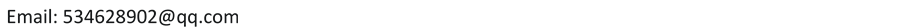Received: Jan. 6th, 2016; accepted: Jan. 25th, 2016; published: Jan. 29th, 2016ABSTRACT

In this paper, we use RKM and ADM decomposition method to solve a class of second-order boundary value problems for integral-differential equations. This method avoids the series solution of the equation with unknown parameters. At the same time, the problem of convergence analysis is also given in this paper. Additionally, some numerical examples are presented to demonstrate the rationality of this algorithm.

Keywords:Two-Order Integral-Differential Equations, Reproducing Kernel Method, ADM Decomposition Method, Convergence Analysis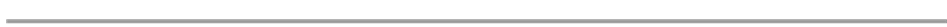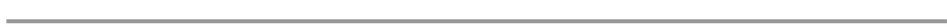1. 引言(1.1)。 (1.2)(1.3)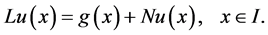(1.4)的逆算子定义为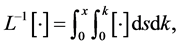(1.5)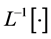作用在(1.4)的两边，并且用用条件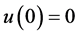，我们可以得到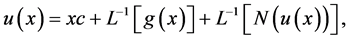(1.6)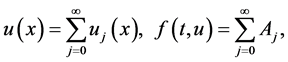(1.7)(1.8)(1.9)(1.10)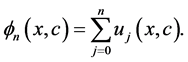(1.11)

2. 新的方法(2.1)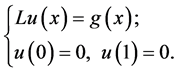(2.2)(2.3)，再生核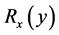定义为(2.4)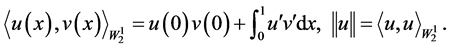(2.5)(2.6)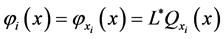(2.7)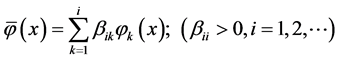(2.8)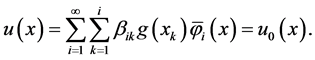(2.9)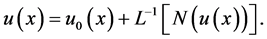(2.10)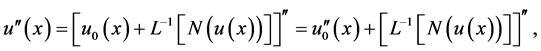(2.11)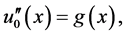(2.12)(2.13)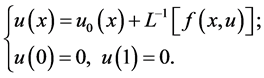(2.14)(2.15)(2.16)

(2.16)给出了方程(1.3)的最终形式，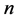次截断得到的级数解为。 (2.17)

3. 收敛性分析(3.1)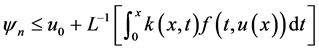。 (3.2)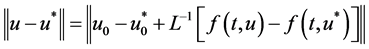，(3.3)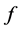满足Lipschitz 条件，并且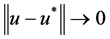，得到， (3.4)， (3.5)(3.6)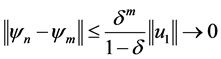， (3.7)

4. 数值算例(4.1)(4.2)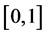上选择6个节点进行计算。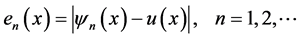(4.3)Table 1. Comparison of the absolute error of Example 4.1 by using new method and modified ADM decomposition method in the paper 

5. 结论

RKM and ADM Decomposition Method for Solving a Class of Two-Order Integral-Differential Equations[J]. 理论数学, 2016, 06(01): 65-71. http://dx.doi.org/10.12677/PM.2016.61010

1. 1. Rach, R.C., Duan, J.S. and Wazwaz, A.M. (2014) Solving Cupled Lane-Emden Boundary Value Problems in Catalytic Diffusion by the Adomian Decomposition Method. Journal of Mathematical Chemistry, 52, 255-267. http://dx.doi.org/10.1007/s10910-013-0260-6

2. 2. Lima, P.M. and Morgado, L. (2010) Numerical Modeling of Oxygen Diffusion in Cells with Michaelis-Menten Up-Take Kinetics. Journal of Mathematical Chemistry, 48, 145-158. http://dx.doi.org/10.1007/s10910-009-9646-x

3. 3. Maleknejad, K., Khalisaraye, I.N. and Alizadeh, M. (2014) On the Solution of the Integro-Differential Equation with an Integral Boundary Condition. Numerical Algorithms, 65, 355-374. http://dx.doi.org/10.1007/s11075-013-9709-8

4. 4. Yao, H. (2010) New Algorithm for the Numerical Solution of the Integro-Differential Equation with an Integral Boundary Condition. Numerical Algorithms, 47, 1054-1067.

5. 5. Cui, M. and Lin, Y. (2008) Nonlinear Numerical Analysis in the Reproducing Kernel Space. Nova Science Publisher, New York.

6. 6. Lv, X. and Shi, S. (2012) The Combined RKM and ADM for Solving Nonlinear Weakly Singular Volterra Integral-Differential Equations. Abstract and Applied Analysis, 2012, Article ID: 258067.

7. 7. Singh, R., Nelakanti, G. and Kumar, J. (2014) A New Efficient Technique for Solving Two-Point Boundary Value Problems for Integro-Differential Equations. Journal of Mathematical Chemistry, 52, 2030-2051. http://dx.doi.org/10.1007/s10910-014-0363-8